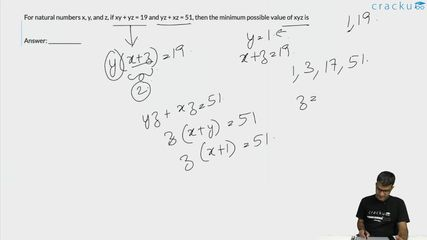Question 63

# For natural numbers x, y, and z, if xy + yz = 19 and yz + xz = 51, then the minimum possible value of xyz is

Solution

It is given, y(x + z) = 19

y cannot be 19.

If y = 19, x + z = 1 which is not possible when both x and z are natural numbers.

Therefore, y = 1 and x + z = 19

It is given, z(x + y) = 51

z can take values 3 and 17

Case 1:

If z = 3, y = 1 and x = 16

xyz = 3*1*16 = 48

Case 2:

If z = 17, y = 1 and x = 2

xyz = 17*1*2 = 34

Minimum value xyz can take is 34.

### View Video Solution• All Quant CAT Formulas and shortcuts PDF
• 30+ CAT previous papers with solutions PDF